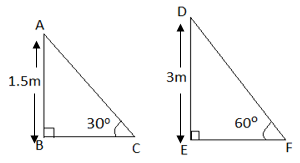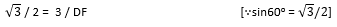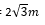Question 3

# A contractor plans to install two slides for the children to play in a park. For the children below the age of 5 years, she prefers to have a slide whose top is at a height of 1.5 m, andis inclined at an angle of 30° to the ground, whereas for elder children, she wants to have a steep slide at a height of 3m, and inclined at an angle of 60° to the ground. What should be the length of the slide in each case?

(i) For Children below age of 5 years

Height of slide = 1.5 m

Angle of inclination of slide = 30o

(ii) For Children below age of 5 years

Height of slide = 3m

Angle of inclination of slide = 60o

Draw figure for both cases:In right Δ ABC, we have

Sin 30o  =  AB / AC           =>     1/ 2  = 1.5 /AC                                                      [ sin30o =1/2]

AC  =  3m

In right Δ DEF, we have

Sin 60o  = DE /DF                 =>DF  =